# Find the RMS value of current I

## Homework Statement

In the network of sinusoidal current, as shown in the figure, the current i2
is phase delayed for the angle of 3π/4 behind the current i.
In the moments in which the current i2 is minimal,
current value of current i1 is sqrt(2) A. This value is two times lower than the maximum
value of the current i1, and in those moments current i1
and growing.
Calculate the effective value of the current I.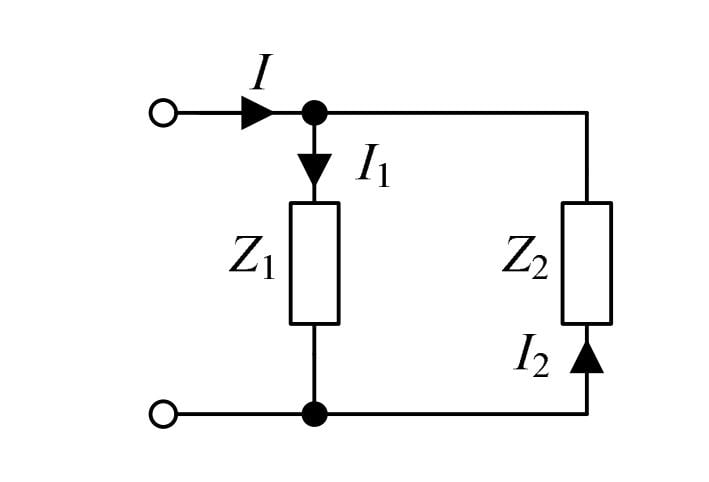[/PLAIN] [Broken]

## The Attempt at a Solution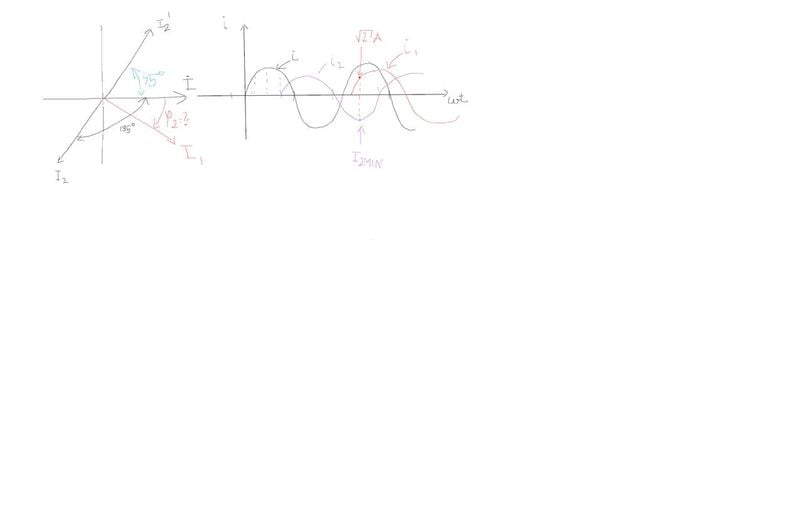capture screen
[/B]
Is there any way to find the angle Φ2 since I know that in the moments when i2 is minimal value of the current i1 is sqrt(2)?

Last edited by a moderator:

rude man
Homework Helper
Gold Member
Did you ask what "effective current" means? I couldn't even guess.

Did you ask what "effective current" means? I couldn't even guess.
It's RMS value( Imax/ sqrt(2) ) .

I tried to solve it this way now:
i = Imax *sin(wt)
i1 = I1max *sin(wt + ψ1)
i2 = I2max *sin(wt+ 3*pi/4 )

at time t = t1 when current i2 is minimal.
-I2max = I2max * sin(w*t1 + 3*pi/4) -------> wt1 = -5*pi /4
2*i(t1) = I1max ------> I1max = 2*sqrt(2)

sqrt(2) = 2sqrt(2) * sin (w*t2 + ψ1) / arcsin
ψ1 = 17*pi / 12

which is incorrect the the correct phase delay of the current i1 is ψ1 = 45°

rude man
Homework Helper
Gold Member

## Homework Statement

In the network of sinusoidal current, as shown in the figure, the current i2
is phase delayed for the angle of 3π/4 behind the current i.
In the moments in which the current i2 is minimal,
current value of current i1 is sqrt(2) A.
What is A? It's not related to anything else in the problem.
and in those moments current i1
and growing.
???
Totally confusing problem statement.

NascentOxygen
Staff Emeritus
the current i2
is phase delayed for the angle of 3π/4 behind the current i.
In the moments in which the current i2 is minimal,
current value of current i1 is sqrt(2) A. This value is two times lower than the maximum
value of the current i1, and in those moments current i1
and growing.
By "is minimal" for a sinusoid should we understand it to indicate a zero-crossing or a negative peak?

NascentOxygen
Staff Emeritus
What is A? It's not related to anything else in the problem.???
A is the abbreviation of "amperes".

NascentOxygen
Staff Emeritus
I tried to solve it this way now:
i = Imax *sin(wt)
i1 = I1max *sin(wt + ψ1)
i2 = I2max *sin(wt+ 3*pi/4 )
i2 is delayed, so phase should be negative.
at time t = t1 when current i2 is minimal.
-I2max = I2max * sin(w*t1 + 3*pi/4) -------> wt1 = -5*pi /4
2*i(t1) = I1max ------> I1max = 2*sqrt(2)

sqrt(2) = 2sqrt(2) * sin (w*t2 + ψ1) / arcsin
ψ1 = 17*pi / 12

which is incorrect the the correct phase delay of the current i1 is ψ1 = 45°

•Ivan Antunovic
Sorry for the late replay guys,I had exam this week.
By "is minimal" for a sinusoid should we understand it to indicate a zero-crossing or a negative peak?
I think that they mean for minimal as value when it is a negative peak of the current.
A is the abbreviation of "amperes".
Correct.
i2 is delayed, so phase should be negative.
Tried calculations now with negative sign of ψ2 = -3π/ 4 and the result that I got is ψ1 = -π / 12 , which gives ψ1 - ψ2 = 2π/3 as it is correct.Now need to figure out how to find RMS value of the current I.

Last edited:
Got the right solution now: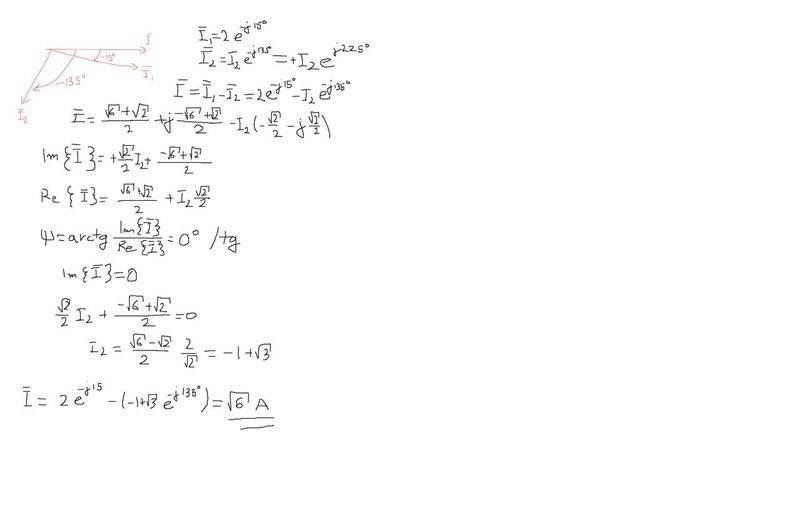Their solution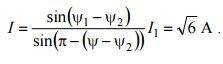How did they get this expression ? It looks way simpler than my approach.

NascentOxygen
Staff Emeritus
Looks like they applied The Sine Rule. The 3 currents form a closed triangle.

•Ivan Antunovic
Looks like they applied The Sine Rule. The 3 currents form a closed triangle.
Yes you are right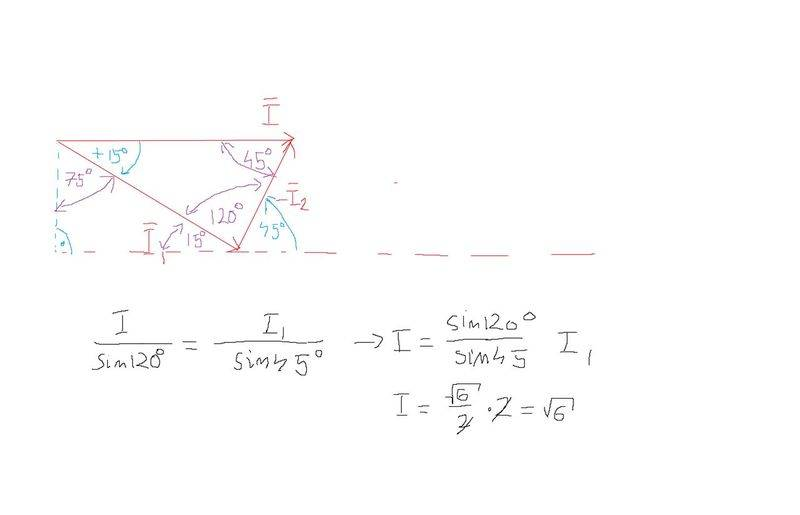•